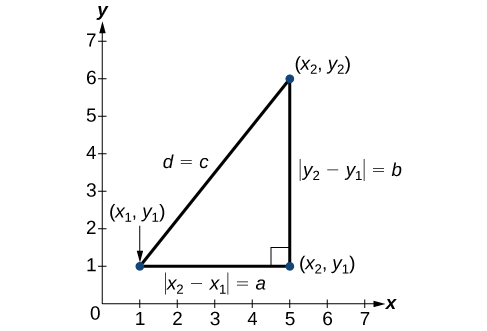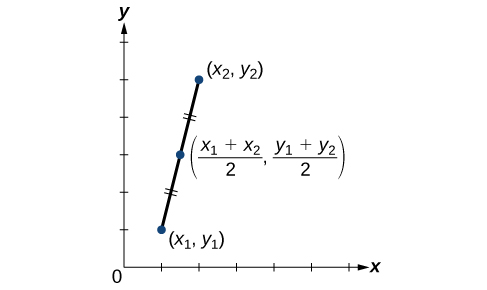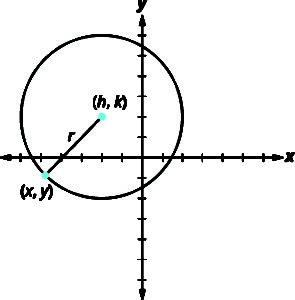## 13.1 – Distance Formula, Midpoint Formula, and Circles

### Learning Objectives

• (13.1.1) – Using the distance formula
• (13.1.2) – Using the midpoint formula
• (13.1.3) – Using the standard form of equations of circles

# (13.1.1) – Using the distance formula

Derived from the Pythagorean Theorem, the distance formula is used to find the distance between two points in the plane. The Pythagorean Theorem, ${a}^{2}+{b}^{2}={c}^{2}$, is based on a right triangle where a and b are the lengths of the legs adjacent to the right angle, and c is the length of the hypotenuse.The relationship of sides $|{x}_{2}-{x}_{1}|$ and $|{y}_{2}-{y}_{1}|$ to side d is the same as that of sides a and b to side c. We use the absolute value symbol to indicate that the length is a positive number because the absolute value of any number is positive. (For example, $|-3|=3$. ) The symbols $|{x}_{2}-{x}_{1}|$ and $|{y}_{2}-{y}_{1}|$ indicate that the lengths of the sides of the triangle are positive. To find the length c, take the square root of both sides of the Pythagorean Theorem.

${c}^{2}={a}^{2}+{b}^{2}\rightarrow c=\sqrt{{a}^{2}+{b}^{2}}$

It follows that the distance formula is given as

${d}^{2}={\left({x}_{2}-{x}_{1}\right)}^{2}+{\left({y}_{2}-{y}_{1}\right)}^{2}\to d=\sqrt{{\left({x}_{2}-{x}_{1}\right)}^{2}+{\left({y}_{2}-{y}_{1}\right)}^{2}}$

We do not have to use the absolute value symbols in this definition because any number squared is positive.

### A General Note: The Distance Formula

Given endpoints $\left({x}_{1},{y}_{1}\right)$ and $\left({x}_{2},{y}_{2}\right)$, the distance between two points is given by

$d=\sqrt{{\left({x}_{2}-{x}_{1}\right)}^{2}+{\left({y}_{2}-{y}_{1}\right)}^{2}}$

### Example: Finding the Distance between Two Points

Find the distance between the points $\left(-3,-1\right)$ and $\left(2,3\right)$.

### Try It

Find the distance between two points: $\left(1,4\right)$ and $\left(11,9\right)$.

### Example: Finding the Distance between Two Locations

Let’s return to the situation introduced at the beginning of this section.

Tracie set out from Elmhurst, IL, to go to Franklin Park. On the way, she made a few stops to do errands. Each stop is indicated by a red dot. Find the total distance that Tracie traveled. Compare this with the distance between her starting and final positions.

# (13.1.2) – Using the midpoint formula

When the endpoints of a line segment are known, we can find the point midway between them. This point is known as the midpoint and the formula is known as the midpoint formula. Given the endpoints of a line segment, $\left({x}_{1},{y}_{1}\right)$ and $\left({x}_{2},{y}_{2}\right)$, the midpoint formula states how to find the coordinates of the midpoint $M$.

$\displaystyle M=\left(\frac{{x}_{1}+{x}_{2}}{2},\frac{{y}_{1}+{y}_{2}}{2}\right)$

A graphical view of a midpoint is shown below. Notice that the line segments on either side of the midpoint are congruent.### Example: Finding the Midpoint of the Line Segment

Find the midpoint of the line segment with the endpoints $\left(7,-2\right)$ and $\left(9,5\right)$.

### Try It

Find the midpoint of the line segment with endpoints $\left(-2,-1\right)$ and $\left(-8,6\right)$.

# (13.1.3) – Using the standard form of equations of circles

CIRCLES

A circle is all points in a plane that are a fixed distance from a given point in the plane. The given point is called the center, $(h,k)$ and the fixed distance is called the radius, $r$, of the circle.

We look at a circle in the rectangular coordinate system. The radius is the distance from the center, $(h,k)$, to a point on the circle, $(x,y)$.To derive the equation of a circle, we can use the distance formula with the points $(h,k)$ and $(x,y)$ and the distance, $r$.

$r=\sqrt{(x-h)^2+(y-k)^2}$

Square both sides:

$r^2 = (x-h)^2+(y-k)^2$.

This gives us the standard form of the equation of the circle of radius $r$ and center $(h,k)$.

### Standard form of the equation of a circle

The standard form of the equation of a circle with center, $(h,k)$ and radius, $r$, is:

$r^2 = (x-h)^2+(y-k)^2$

### Example: Finding the Center of a Circle

The diameter of a circle has endpoints $\left(-1,-4\right)$ and $\left(5,-4\right)$. Find the center of the circle.